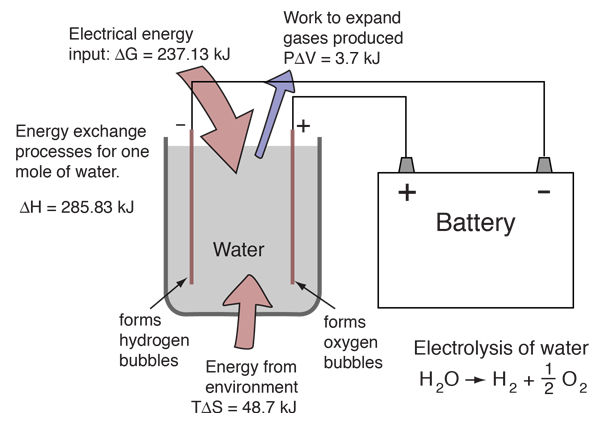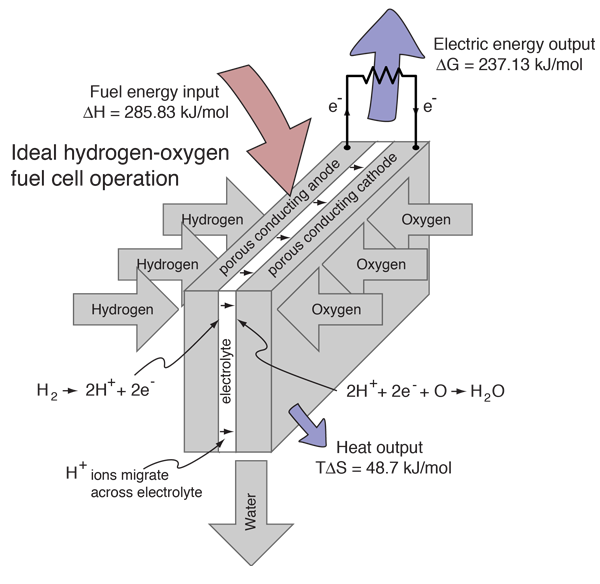# Electrolysis of Water

By providing energy from a battery, water (H2O) can be dissociated into the diatomic molecules of hydrogen (H2) and oxygen (O2). This process is a good example of the the application of the four thermodynamic potentials.The electrolysis of one mole of water produces a mole of hydrogen gas and a half-mole of oxygen gas in their normal diatomic forms. A detailed analysis of the process makes use of the thermodyamic potentials and the first law of thermodynamics. This process is presumed to be at 298K and one atmosphere pressure, and the relevant values are taken from a table of thermodynamic properties.

 Quantity H2O H2 0.5 O2 Change Enthalpy -285.83 kJ 0 0 ΔH = 285.83 kJ Entropy 69.91 J/K 130.68 J/K 0.5 x 205.14 J/K TΔS = 48.7 kJ

The process must provide the energy for the dissociation plus the energy to expand the produced gases. Both of those are included in the change in enthalpy included in the table above. At temperature 298K and one atmosphere pressure, the system work is

W = PΔV = (101.3 x 103 Pa)(1.5 moles)(22.4 x 10-3 m3/mol)(298K/273K) = 3715 J

Since the enthalpy H= U+PV, the change in internal energy U is then

ΔU = ΔH - PΔV = 285.83 kJ - 3.72 kJ = 282.1 kJ

This change in internal energy must be accompanied by the expansion of the gases produced, so the change in enthalpy represents the necessary energy to accomplish the electrolysis. However, it is not necessary to put in this whole amount in the form of electrical energy. Since the entropy increases in the process of dissociation, the amount TΔS can be provided from the environment at temperature T. The amount which must be supplied by the battery is actually the change in the Gibbs free energy:

ΔG = ΔH - TΔS = 285.83 kJ - 48.7 kJ = 237.1 kJ

Since the electrolysis process results in an increase in entropy, the environment "helps" the process by contributing the amount TΔS. The utility of the Gibbs free energy is that it tells you what amount of energy in other forms must be supplied to get the process to proceed.

 Reverse process: Hydrogen fuel cell
Index

Internal energy concepts

Electrochemistry concepts

Reference
Schroeder
Ch 5

 HyperPhysics***** Thermodynamics R Nave
Go Back

# Hydrogen Fuel Cell

Hydrogen and oxygen can be combined in a fuel cell to produce electrical energy. A fuel cell uses a chemical reaction to provide an external voltage, as does a battery, but differs from a battery in that the fuel is continually supplied in the form of hydrogen and oxygen gas. It can produce electrical energy at a higher efficiency than just burning the hydrogen to produce heat to drive a generator because it is not subject to the thermal bottleneck from the second law of thermodynamics. It's only product is water, so it is pollution-free. All these features have led to periodic great excitement about its potential, but we are still in the process of developing that potential as a pollution-free, efficient energy source (see Kartha and Grimes).

Note that when the descriptor "efficient energy source" is used here, it just refers to the relative efficiency with which the energy is applied to a task, not that it is the ultimate source of that energy. The fuel cell does not generate energy, but just transforms the energy contained in the hydrogen and oxygen fuel to a useful electrical energy output. The overall practicality of fuel cells depends upon the efficiency of the process of producing the hydrogen and oxygen fuels which power the cells. Getting the hydrogen and oxygen fuels from other, more primary, sources of energy has proved to be the limitation on the application of fuel cells. If solar or wind energy could be efficiently used to electrolyze water, the practicality of fuel cells would be enhanced.Combining a mole of hydrogen gas and a half-mole of oxygen gas from their normal diatomic forms produces a mole of water. A detailed analysis of the process makes use of the thermodynamic potentials. This process is presumed to be at 298K and one atmosphere pressure, and the relevant values are taken from a table of thermodynamic properties.

 Quantity H2 0.5 O2 H2O Change Enthalpy 0 0 -285.83 kJ ΔH = -285.83 kJ Entropy 130.68 J/K 0.5 x 205.14 J/K 69.91 J/K TΔS = -48.7 kJ

Energy is provided by the combining of the atoms and from the decrease of the volume of the gases. Both of those are included in the change in enthalpy included in the table above. At temperature 298K and one atmosphere pressure, the system work is

W = PΔV = (101.3 x 103 Pa)(1.5 moles)(-22.4 x 10-3 m3/mol)(298K/273K) = -3715 J

Since the enthalpy H= U+PV, the change in internal energy U is then

ΔU = ΔH - PΔV = -285.83 kJ - 3.72 kJ = -282.1 kJ

The entropy of the gases decreases by 48.7 kJ in the process of combination since the number of water molecules is less than the number of hydrogen and oxygen molecules combining. Since the total entropy will not decrease in the reaction, the excess entropy in the amount TΔS must be expelled to the environment as heat at temperature T. The amount of energy per mole of hydrogen which can be provided as electrical energy is the change in the Gibbs free energy:

ΔG = ΔH - TΔS = -285.83 kJ + 48.7 kJ = -237.1 kJ

For this ideal case, the fuel energy is converted to electrical energy at an efficiency of 237.1/285.8 x100% = 83%! This is far greater than the ideal efficiency of a generating facility which burned the hydrogen and used the heat to power a generator! Although real fuel cells do not approach that ideal efficiency, they are still much more efficient than any electric power plant which burns a fuel.

Comparison of electrolysis and the fuel cell process

In comparing the fuel cell process to its reverse reaction, electrolysis of water, it is useful treat the enthalpy change as the overall energy change. The Gibbs free energy is that which you actually have to supply if you want to drive a reaction, or the amount that you can actually get out if the reaction is working for you. So in the electrolysis/fuel cell pair where the enthalpy change is 285.8 kJ, you have to put in 237 kJ of energy to drive electrolysis and the heat from the environment will contribute TΔS=48.7 kJ to help you. Going the other way in the fuel cell, you can get out the 237 kJ as electric energy, but have to dump TΔS = 48.7 kJ to the environment.

Index

Internal energy concepts

Electrochemistry concepts

Reference
Schroeder
Ch 5

Reference
Kartha & Grimes

 HyperPhysics***** Thermodynamics R Nave
Go Back